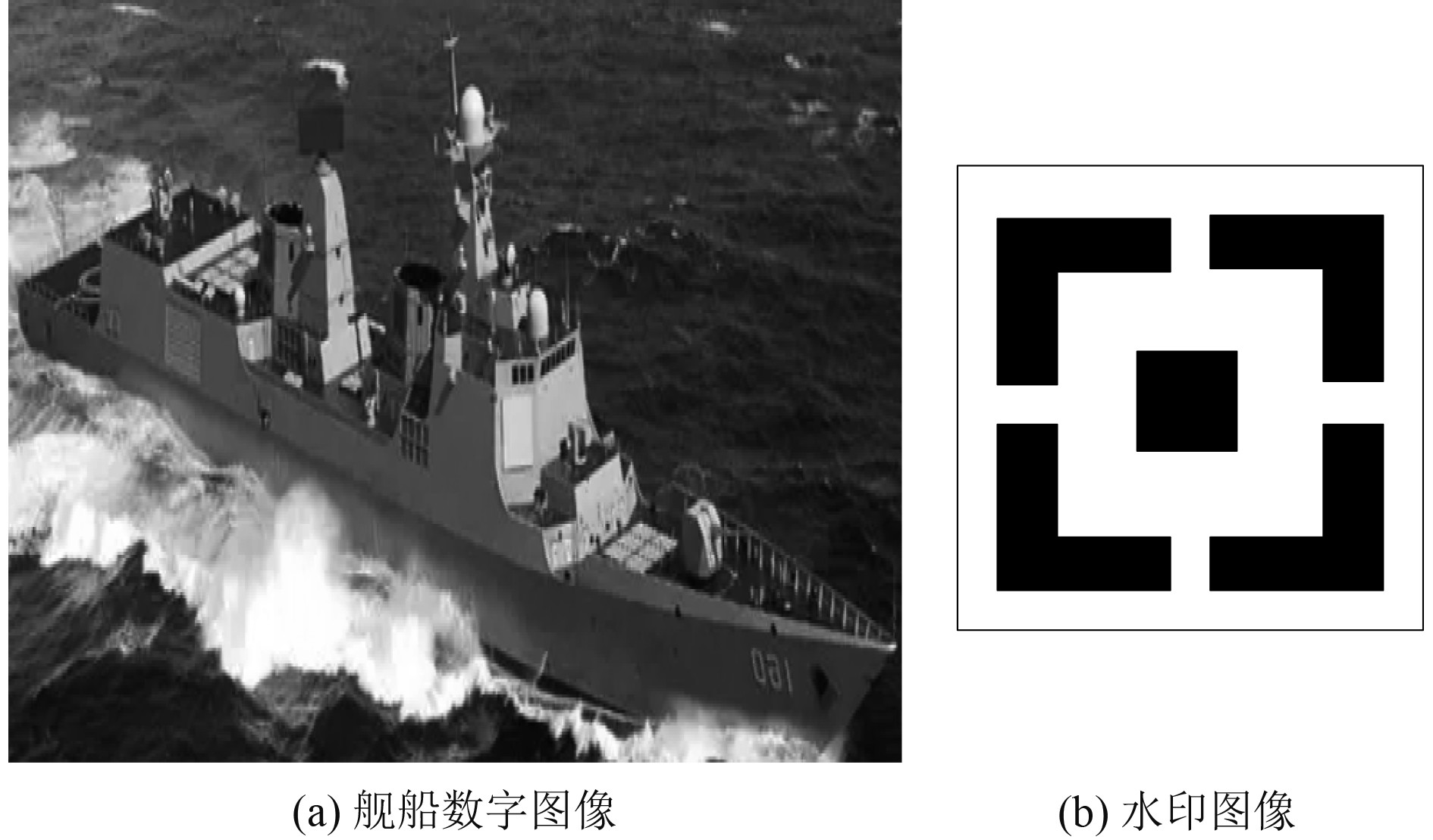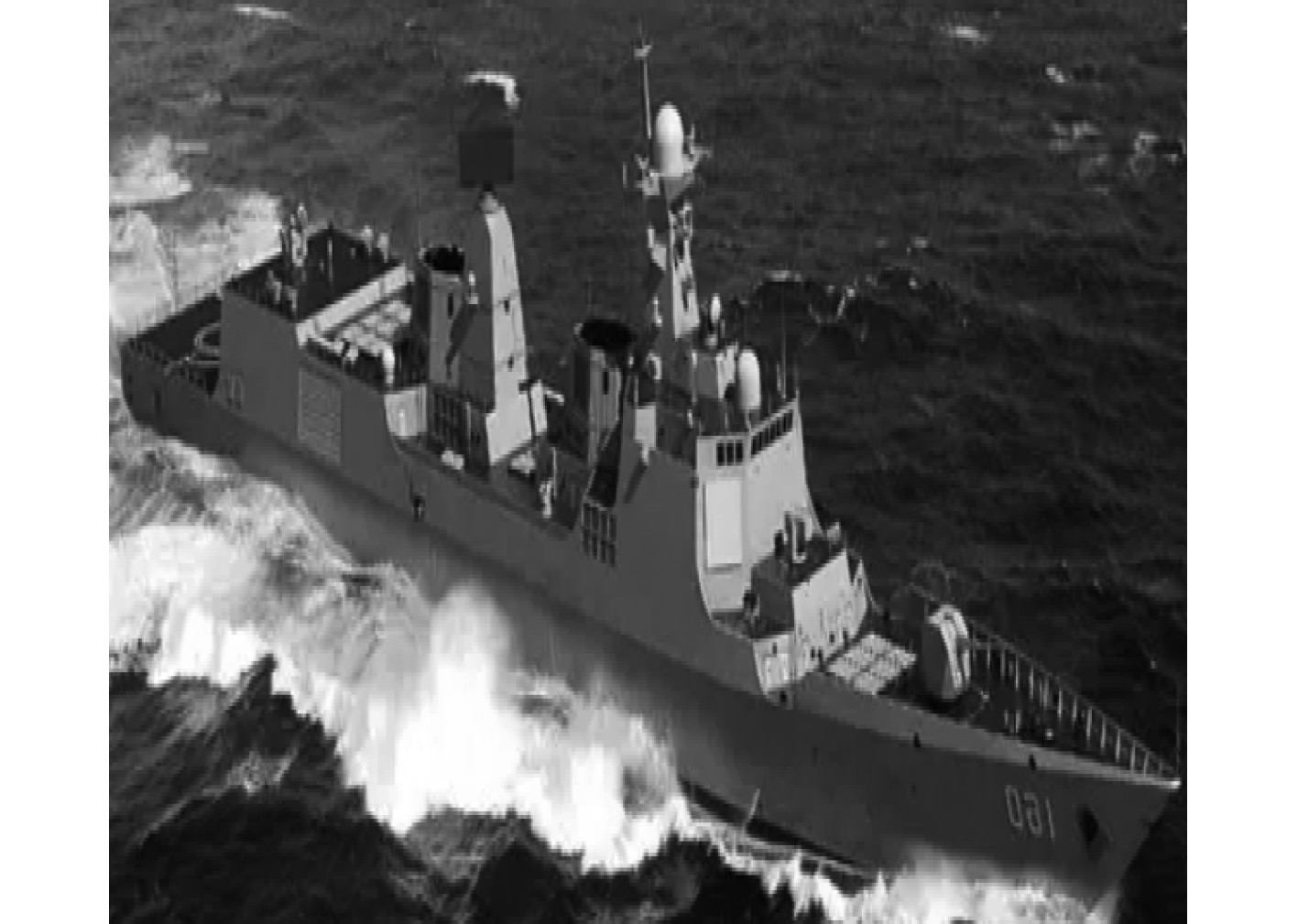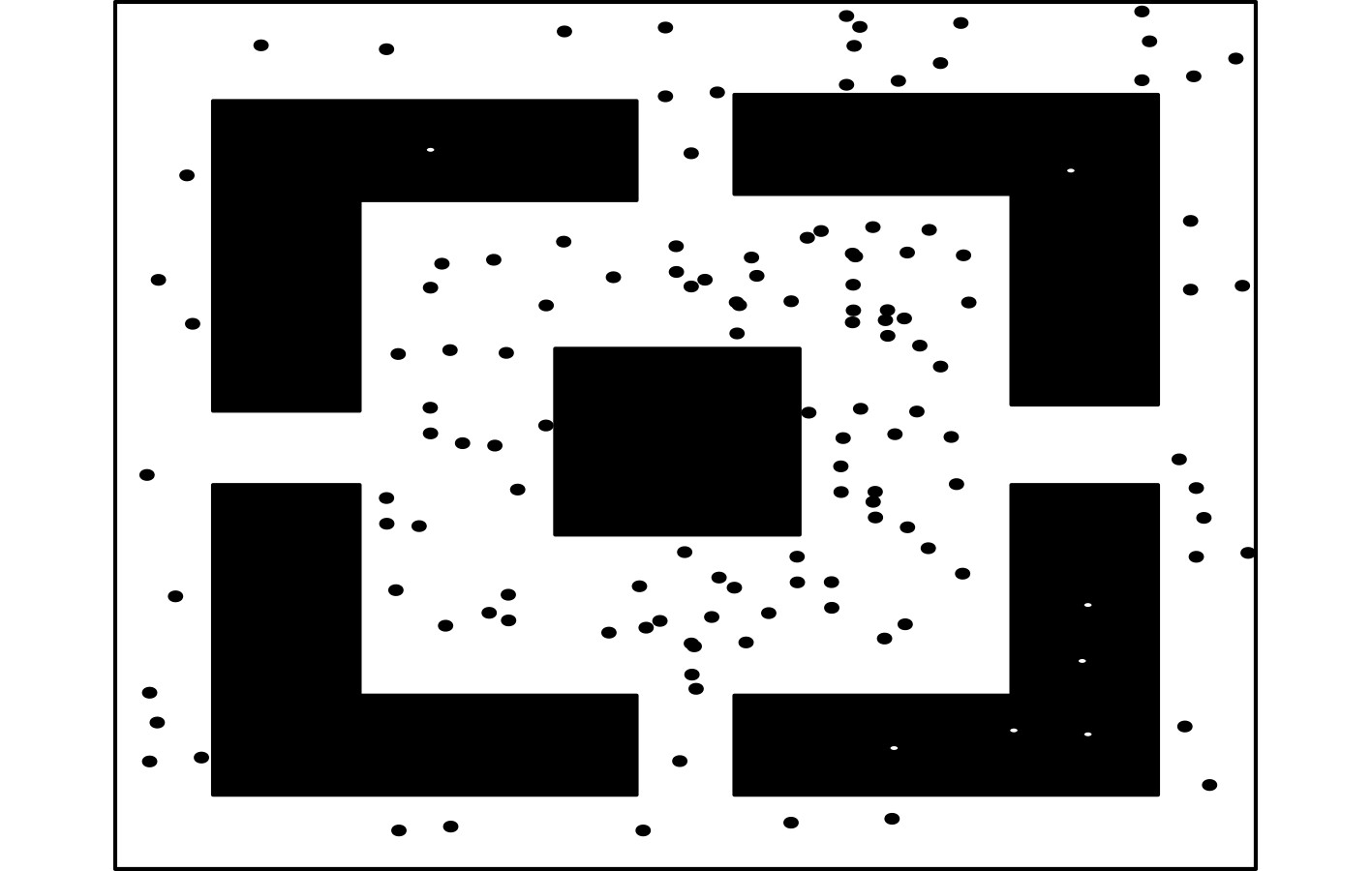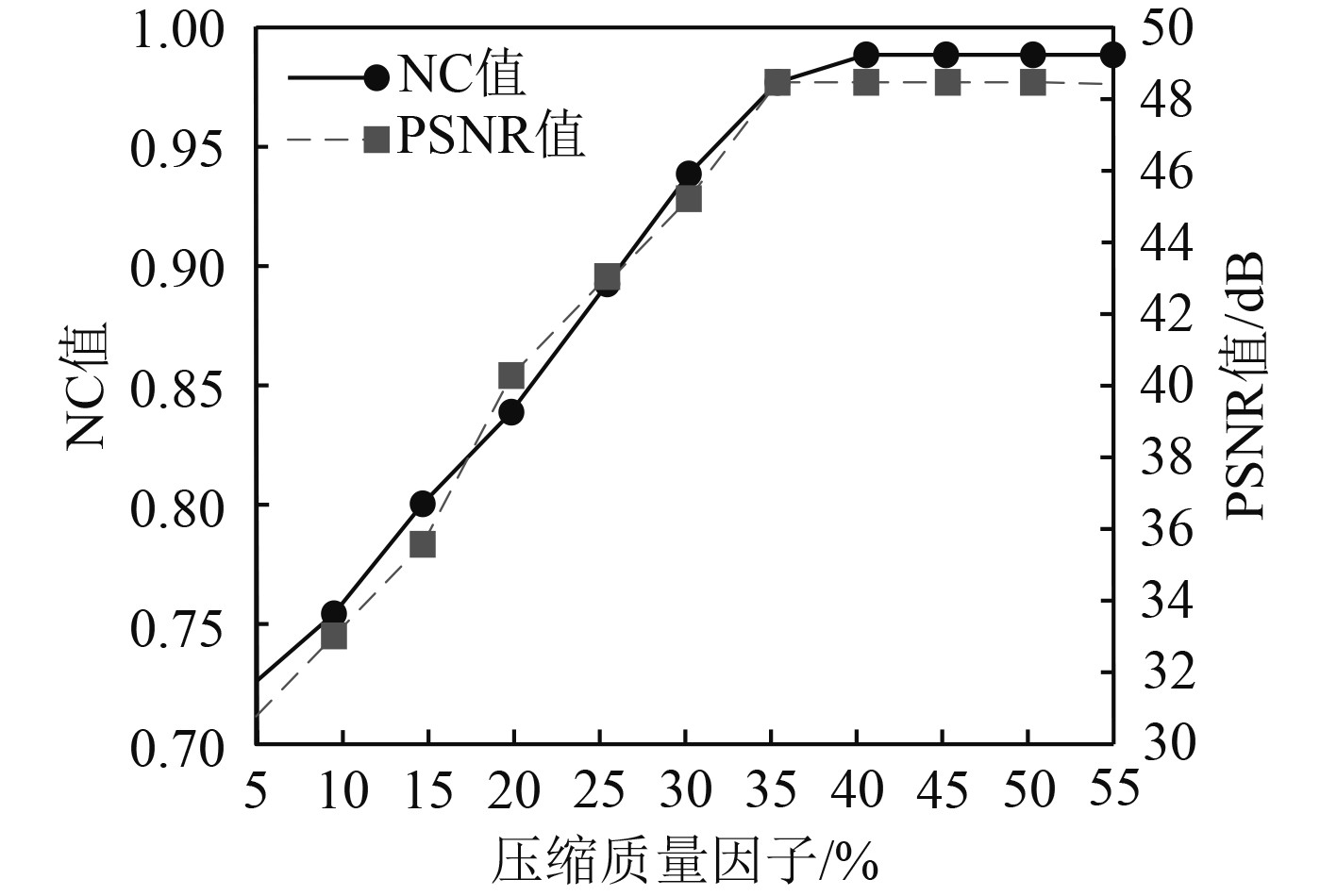﻿ 改进压缩感知的舰船数字图像水印算法
 舰船科学技术2022, Vol. 44Issue (19): 166-169    DOI: 10.3404/j.issn.1672-7649.2022.19.034PDF

Watermarks for ship digital images based on improved compression sensing
XU Pei, SHA Chang-tao
China Electronice Standardization Institute, Beijing 100176, China
Abstract: In order to improve the embedding and extraction effect of digital image watermarking, an improved ship digital image watermarking algorithm based on compressed sensing is studied. The high frequency and low frequency coefficient matrices are obtained by sparse decomposition of ship digital images by discrete wavelet transform. Using Kent chaotic observation matrix to improve compressed sensing theory. The observation values of high and low frequency coefficient matrices are obtained according to the improved compressed sensing theory. The watermark is embedded in the observed value of the high frequency coefficient matrix, and the embedding position is recorded to establish the index matrix. Through the improved orthogonal matching pursuit algorithm of compressed sensing reconstruction, the observed values of high and low frequency coefficient matrices embedded in the watermark were reconstructed, and the high and low frequency coefficient matrices were obtained. The high and low frequency coefficient matrices are fused, and the fused matrix is processed by inverse discrete wavelet transform, and the watermark embedded ship digital image is obtained. Using index matrix and observation matrix of high frequency coefficient matrix, the watermark is extracted. Experimental results show that the algorithm can effectively embed and extract ship digital image watermarks. Under different attack types, the transparency of watermark embedding is better, the precision of watermark extraction is higher, and the algorithm has better anti-rotation and anti-shear attack ability.
Key words: improve compression awareness     digital image of ship     high frequency coefficient     wavelet transform     Kent chaos     orthogonal matching
0 引　言

1 舰船数字图像水印算法

1.1 基于DWT的舰船数字图像稀疏分解

 ${\psi _{m,n}}\left( t \right) = \dfrac{{\psi \left( {\dfrac{{t - nba_{}^m}}{{a_{}^m}}} \right)}}{{\sqrt {a_{}^m} }}。$ (1)

 ${W_f}\left( {m,n} \right) = \int_R {f\left( t \right){\psi _{m,n}}\left( t \right){\rm{d}}t}。$ (2)

 ${X'_1} = \sum\limits_{z = - \infty }^\infty {{W_f}X\left( z \right)h\left( {m - z} \right)}。$ (3)

 ${\hat X_1} = \sum\limits_{z = - \infty }^\infty {{W_f}X\left( z \right)h\left( {2m - z} \right)}，$ (4)

 ${\hat X_2} = \sum\limits_{z = - \infty }^\infty {{W_f}X\left( z \right)g\left( {2m - z} \right)}。$ (5)

1.2 基于改进压缩感知的舰船数字图像水印算法的实现

 ${y_1} = {\boldsymbol{\varphi}} \theta 。$ (6)

 ${s_1} = {{\boldsymbol{\varPhi}} _1}{\boldsymbol{\varphi}} \theta = {{\boldsymbol{Q}}_1}\theta，$ (7)

Kent混沌映射为离散混沌系统，且具备代表性，表达公式如下：

 ${\hat x_{1,l + 1}} = \left\{ \begin{array}{*{20}{l}} \dfrac{{{{\hat x}_{1,l}}}}{v},& 0 < {{\hat x}_{1,l}} \leqslant v ，\\ \left( {1 - {{\hat x}_{1,l}}} \right)\left( {1 - v} \right),& v< {{\hat x}_{1,l}} < 1 。\\ \end{array} \right.$ (8)

 ${\hat {\boldsymbol{\varPhi}} _1} = \frac{1}{{\sqrt M \cdot \sigma \cdot \lambda }}\left( \begin{array}{*{20}{c}} {{\hat x}_{1,0}}&{{\hat x}_{1,M}}& \cdots & {{\hat x}_{1,M\left( {L - 1} \right)}} \\ {{\hat x}_{1,1}}&{{\hat x}_{1,M + 1}}&\cdots& {{\hat x}_{1,M\left( {L - 1} \right) + 1}} \\ { \vdots}& \vdots& \cdots& \vdots \\ {{\hat x}_{1,M - 1}}& {{\hat x}_{1,2M - 1}}& \cdots&{{\hat x}_{1,ML - 1}} \end{array} \right)。$ (9)

 $\left[ \begin{gathered} {p'} \\ {q'} \\ \end{gathered} \right] = \left[ \begin{gathered} 1\mathop {}\nolimits_{} 1 \\ 1\mathop {}\nolimits_{} 2 \\ \end{gathered} \right]\left[ \begin{gathered} p \\ q \\ \end{gathered} \right]\bmod \rho。$ (10)

 ${s'_{1,2\tau }} = \left\{ \begin{array}{*{20}{l}} {s_{1,2\tau - 1}} + \alpha \varsigma \varpi ,& \left| {{s_{1,2\tau }} - {s_{1,2\tau - 1}}} \right| < \varepsilon ' ，\\ {s_{1,2\tau }},& {\rm{else}} 。\ \end{array} \right.$ (11)

2 实验分析图 1 舰船数字图像与水印图像 Fig. 1 Ship Digital Image and Watermark Image图 2 嵌入水印后的舰船数字图像 Fig. 2 Digital image of ship embedded with watermark图 3 水印提取结果 Fig. 3 Watermark extraction results图 4 舰船数字图像水印嵌入与提取效果 Fig. 4 Watermark embedding and extraction effect of ship digital image

3 结　语

  李伟岸, 熊祥光, 夏道勋. 基于Schur分解和混沌置乱的彩色图像鲁棒水印算法[J]. 计算机工程与科学, 2021, 43(7): 1243-1249. DOI:10.3969/j.issn.1007-130X.2021.07.013  牛盼盼, 王向阳, 杨思宇, 等. 基于二元Weibull分布的非下采样Shearlet域图像水印算法[J]. 计算机研究与发展, 2019, 56(7): 1454-1469. DOI:10.7544/issn1000-1239.2019.20180278  齐向明, 徐嫚, 李玥, 等. 一种尺度空间特征区域的强鲁棒性水印算法[J]. 计算机应用研究, 2019, 36(6): 1793-1796+1802. DOI:10.19734/j.issn.1001-3695.2018.01.0056  沈艳冰, 杨阳, 李竹. 一种适用于QR码的彩色图像数字水印算法[J]. 智能系统学报, 2020, 15(5): 949-955. DOI:10.11992/tis.201903016  李淑芝, 龙香玉, 邓小鸿, 等. 结合纹理复杂度和JND模型的图像水印算法[J]. 计算机应用研究, 2019, 36(2): 528-531+542. DOI:10.19734/j.issn.1001-3695.2017.09.0900  王楠, 李智, 程欣宇, 等. 基于均值比例与压缩感知的视频双水印算法[J]. 计算机工程, 2019, 45(3): 250-255+261. DOI:10.19678/j.issn.1000-3428.0052206  李莹莹, 张毅锋, 程旭, 等. 基于DWT最优多子图和SIFT几何校正的鲁棒水印算法[J]. 计算机应用研究, 2019, 36(6): 1819-1823+1827. DOI:10.19734/j.issn.1001-3695.2018.01.0042  刘琰, 周理. 基于小波变换域的数字图像嵌入和提取方法[J]. 沈阳工业大学学报, 2019, 41(1): 68-72. DOI:10.7688/j.issn.1000-1646.2019.01.13